Fundamentals of Horn Theory

Chapter  4

 The parameter that has the most effect on the physical size of a horn is the low frequency cutoff, fc.  These things will become very big, very fast, as that frequency is lowered.  Even in the super efficient space saving Klipschorn®, a reduction in fc of 10 Hz will result in a horn that is difficult to pass through a doorway.  That's only one of the problems encountered.  If the horn is to operate above about 200 Hz, the radii of curvature around the bends will be will be too large to effectively transmit the acoustical pressure wave and irregular response will result.  In a bass horn operating well below about 200 Hz, this effect will be negligible.

 In dwg. 4.1 we see the throat area, At, the air chamber, V,  behind the diaphragm and the air enclosed in the volume, Vo, between the diaphragm and the throat.  The volume, Vo, as mentioned earlier will have little to no effect below about 200 Hz.  However, above this, it will behave as a low pass filter.  High frequency reflections within the boundaries of this volume will create irregular response, an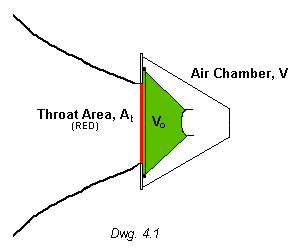undesirable characteristic for high fidelity reproduction.  In today's power hungry society, loudspeakers with heavy diaphragms and 3 to 4 inch voice coils further limit higher frequency response due to what is referred to as mass loading.  The high voice coil inductance and mass of the diaphragm will limit smooth response beyond a few hundred hertz. The air chamber, V, acts to equalize the load on both sides of the diaphragm.  Remember, there IS a column of air in front of the diaphragm in a horn.  An approximation for this rear volume is 3 times the throat area times the length of the horn in which the cross sectional area doubles. V = 3 At l Calculating the throat area is no easy task.  Fortunately, if one has access to the test equipment required to determine the Theile/Small parameters of a loudspeaker, one can easily determine cone mass or more accurately, Mms which is the mass of the moving system.  I refer the reader to Vance Dickason's book titled "The Loudspeaker Design Cookbook".   ISBN 0-9624-191-7-6   That number is from a 1991 edition. For the curious, C.R. Hanna, in a paper (see below) published in 1928 gives the following relationships.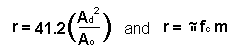In the above equation, Ad  and  Ao are the diaphragm and throat areas, respectively;  r  is the radiation damping due to the horn, fc  is the low frequency cutoff and  m  is,  yup, you guessed it, the mass of the moving system.   The little squiggly thing after the = sign is pi, or 3.14159.........   The general equation of the exponential horn, in it's simplest form, is  given by the equation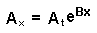which states that the cross sectional area, A, at a distance x from the throat is equal to the throat area, At, multiplied by e, the base of natural logarithms which is 2.71828 raised to the power of B times x.  B is the flare rate constant, in inverse dimensions, be they inches, centimeters or what have you.  The derivation of B comes from the relationship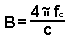where fc  is the cutoff frequency and c is the velocity of sound in air.  The value of c at 25 degrees Centigrade, 76 degrees Fahrenheit is  13,628 inches/sec. or 34,615 cm./sec.     A recently obtained paper gives the throat area, At as follows.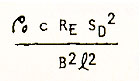where po is the density of air,  1.2 kg/m^3,  c is the velocity of sound in air, 343 m/s, Re is the voice coil dc resistance, SD is the effective piston (cone/diaphragm) area, in square meters and B^2l^2 is the BL product squared, in TM (TESLA METERS). An easier to handle conversion of the above equation using the Thiele/Small parameters is as follows.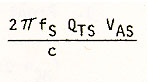The above equations are derived to maximize the efficiency in the midrange passband; they will yield a throat area that will impose an unnecessary load on the diaphragm, i.e., too small for low frequencies.  A good approximation would be to make the throat area about 63% of the effective piston area.  Thus, for a 15 inch speaker, the effective piston area is about 133 in^2 (0.0856m^2) and 63% is 84 in^2.  A rectangular throat measuring 12" by 7" will suffice.The larger throat wi9ll have a small detrimental effect on the low frequency transient response but it'll still be much better than a direct radiator.   It is also much easier to design and build but an alternative exists for the more ambitious builder.  See chapter 6.

 C.R. Hanna  -  Loudspeakers of High Efficiency and Load Capacity.   Presented at the Winter Convention of the A.I.E.E., American Institute of Electrical Engineers, New York, N.Y., February 13-17, 1928.

Chapter 5

Horn Theory - Chapter Index